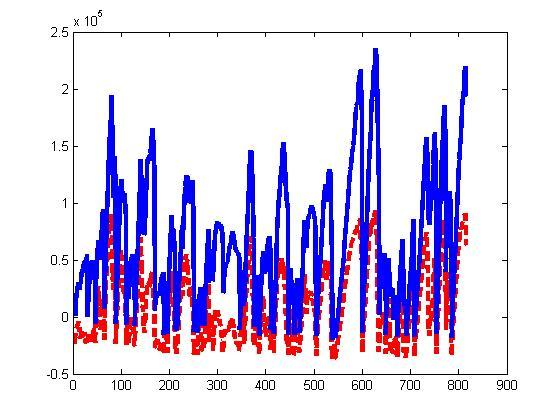# CVX solves the problem, but the inequation constraint is not satisfied

CVX soves my problem, and most of the constraints are satisfied. However, one inequation constraint is almost completely not satisfied, and one is partly satisfied. Why is that? And some of the results seem to be correct. In my problem, a>=b, but the result shows that it is not so.(In the figure, a is the red curve, and b is blue curve)In order to get help, you’ll have to provide more information . Can you post your CVX code, with all input data, which would allow us to see what you’re talking about and assess the situation? I.e., provide us a complete MATLAB session, with all input data, which demonstrates what you’re talking about.

I’m going to guess a scaling issue. But Mark’s right. It is frankly frustrating to me that you would assume we could offer any useful assistance without any information whatsoever about your model or the conditions under which you solved it.

I’ve sent the codes to your email.

Thanks. But I’ve considered the scaling issue. All variables are scaled to [0,1] range.

I ran the code under sedumi and sdpt3.

sedumi reported inaccurate/solved.

sdpt3 reported solved, but had many RCOND warnings on the 44th iteration, which was its last. It had " lack of progress in infeas"

sedumi:

`````` 28 :  -5.85E+02 8.49E-16 0.000 0.3588 0.9000 0.0000   0.99 99 99  5.8E-08
Run into numerical problems.

iter seconds digits       c*x               b*y
28   2578.3   Inf -5.9154857763e+02 -5.8481203788e+02
|Ax-b| =   5.4e-07, [Ay-c]_+ =   6.5E-05, |x|=  4.3e+03, |y|=  6.2e+03

Detailed timing (sec)
Pre          IPM          Post
5.650E-01    4.222E+02    7.201E-02
Max-norms: ||b||=1.616065e-01, ||c|| = 3.794013e+05,
Cholesky |add|=815, |skip| = 0, ||L.L|| = 463.05.
------------------------------------------------------------
Status: Inaccurate/Solved
Optimal value (cvx_optval): +454.005

fuel_consumption =
28.1553
a =
785
>> cvx_status
cvx_status =
Inaccurate/Solved

ans =
0.0592

ans =
-0.3775
ans =
0.0021
``````

sdpt3:

``````44||0.000||0.000||3.0e-10||1.5e-16||1.2e-10||-5.926672e+02 -5.926672e+02|| 0:0:24||
lack of progress in infeas
-------------------------------------------------------------------
number of iterations   = 44
primal objective value = -5.92667221e+02
dual   objective value = -5.92667221e+02
gap := trace(XZ)       = 6.91e-08
relative gap           = 5.82e-11
actual relative gap    = 2.61e-11
rel. primal infeas     = 1.21e-10
rel. dual   infeas     = 4.29e-16
norm(X), norm(y), norm(Z) = 4.3e+03, 6.2e+03, 3.6e+04
norm(A), norm(b), norm(C) = 2.1e+07, 5.6e+00, 2.3e+06
Total CPU time (secs)  = 23.95
CPU time per iteration = 0.54
termination code       =  0
DIMACS: 5.8e-10  0.0e+00  2.6e-15  0.0e+00  2.6e-11  5.8e-11
-------------------------------------------------------------------
------------------------------------------------------------
Status: Solved
Optimal value (cvx_optval): +461.86

fuel_consumption =
28.6425
a =
70
>> cvx_status
cvx_status =
Solved
``````

``````task.b2(ix).*square(Tem2(ix))*St^2)/Sp)
ans =
0.0358
ans =
-0.3736
ans =
0.0334
``````

So it appears that sedumi and sdpt3 both satisfied the first listed inequality, and satisfied the 2nd inequality on only some of the vector inequality elements. Clearly both solvers were encountering some difficulties. It would be interesting to see how MOSEK would do, since it may be more numerically robust.

On my situation, I can run the program under sedumi with best precision but under sdpt3 the matlab would report “out of memory”.
Furthermore,I’ve tried the MOSEK, which seems to calculate faster but remains the same problem.

ans = -0.9597